# Electronics and Communication Engineering - Analog Electronics

6.

In the circuit figure LED will be on when v1 is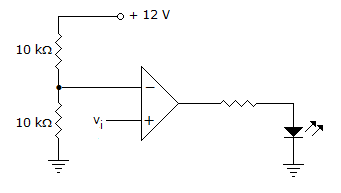A. less than 6 V B. more than 6 V C. less than 12 V D. more than 12 V

Explanation:

Voltage input to inverting input is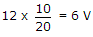. Therefore, vi should be more than 6 V.

7.

A differential amplifier consists of two CB amplifiers.

 A. True B. False

Explanation:

It consists of two CE amplifiers.

8.

Figure shows a portion of linear v - i characteristics of diode. If applied voltage is + 6 V, the current will be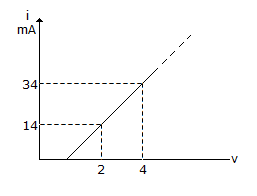A. 42 mA B. 48 mA C. 51 mA D. 54 mA

Explanation: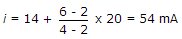.

9.

A power transistor dissipates 5 W. If ambient temperature is 30° and case to air thermal resistance is 10° C/W, the case temperature is

 A. 80°C B. 20°C C. 32°C D. 305°C

Explanation: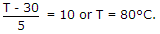10.

An R-C coupled amplifier has mid-frequency gain of 200 and a frequency response from 100 Hz to 20 kHz. A negative feedback network with β = 0.2 is incorporated into the amplifier circuit, the Bandwidth will be

 A. 50 kHz B. 20 kHz C. 100 kHz D. infinite

Explanation: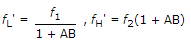B.W. = fH' - fL' .